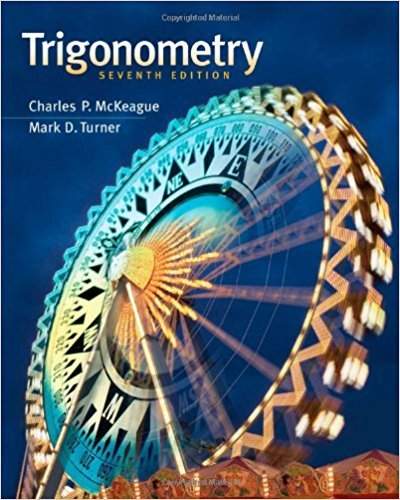×
×

# Solutions for Chapter 3.2: Radians and Degrees## Full solutions for Trigonometry | 7th Edition

ISBN: 9781111826857Solutions for Chapter 3.2: Radians and Degrees

Solutions for Chapter 3.2
4 5 0 299 Reviews
30
3
##### ISBN: 9781111826857

Since 123 problems in chapter 3.2: Radians and Degrees have been answered, more than 26110 students have viewed full step-by-step solutions from this chapter. This expansive textbook survival guide covers the following chapters and their solutions. This textbook survival guide was created for the textbook: Trigonometry, edition: 7. Chapter 3.2: Radians and Degrees includes 123 full step-by-step solutions. Trigonometry was written by and is associated to the ISBN: 9781111826857.

Key Math Terms and definitions covered in this textbook
• Big formula for n by n determinants.

Det(A) is a sum of n! terms. For each term: Multiply one entry from each row and column of A: rows in order 1, ... , nand column order given by a permutation P. Each of the n! P 's has a + or - sign.

• Cayley-Hamilton Theorem.

peA) = det(A - AI) has peA) = zero matrix.

• Column space C (A) =

space of all combinations of the columns of A.

• Cross product u xv in R3:

Vector perpendicular to u and v, length Ilullllvlll sin el = area of parallelogram, u x v = "determinant" of [i j k; UI U2 U3; VI V2 V3].

• Full column rank r = n.

Independent columns, N(A) = {O}, no free variables.

• Full row rank r = m.

Independent rows, at least one solution to Ax = b, column space is all of Rm. Full rank means full column rank or full row rank.

• Hermitian matrix A H = AT = A.

Complex analog a j i = aU of a symmetric matrix.

• Inverse matrix A-I.

Square matrix with A-I A = I and AA-l = I. No inverse if det A = 0 and rank(A) < n and Ax = 0 for a nonzero vector x. The inverses of AB and AT are B-1 A-I and (A-I)T. Cofactor formula (A-l)ij = Cji! detA.

• Markov matrix M.

All mij > 0 and each column sum is 1. Largest eigenvalue A = 1. If mij > 0, the columns of Mk approach the steady state eigenvector M s = s > O.

• Normal matrix.

If N NT = NT N, then N has orthonormal (complex) eigenvectors.

• Nullspace matrix N.

The columns of N are the n - r special solutions to As = O.

• Orthogonal matrix Q.

Square matrix with orthonormal columns, so QT = Q-l. Preserves length and angles, IIQxll = IIxll and (QX)T(Qy) = xTy. AlllAI = 1, with orthogonal eigenvectors. Examples: Rotation, reflection, permutation.

• Orthonormal vectors q 1 , ... , q n·

Dot products are q T q j = 0 if i =1= j and q T q i = 1. The matrix Q with these orthonormal columns has Q T Q = I. If m = n then Q T = Q -1 and q 1 ' ... , q n is an orthonormal basis for Rn : every v = L (v T q j )q j •

• Outer product uv T

= column times row = rank one matrix.

• Polar decomposition A = Q H.

Orthogonal Q times positive (semi)definite H.

• Rank one matrix A = uvT f=. O.

Column and row spaces = lines cu and cv.

• Simplex method for linear programming.

The minimum cost vector x * is found by moving from comer to lower cost comer along the edges of the feasible set (where the constraints Ax = b and x > 0 are satisfied). Minimum cost at a comer!

• Special solutions to As = O.

One free variable is Si = 1, other free variables = o.

• Symmetric factorizations A = LDLT and A = QAQT.

Signs in A = signs in D.

• Vandermonde matrix V.

V c = b gives coefficients of p(x) = Co + ... + Cn_IXn- 1 with P(Xi) = bi. Vij = (Xi)j-I and det V = product of (Xk - Xi) for k > i.

×